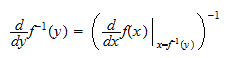StatLect

# Derivatives - Review

This review page contains a summary of differentiation rules, that is, of rules for computing the derivative of a function. Ifis a function, its first derivative is denoted by.## Derivative of a constant function

Ifis a constant functionwhere, then its first derivative is## Derivative of a power function

Ifis a power functionthen its first derivative iswhereis a constant.

## Derivative of a logarithmic function

Ifis the natural logarithm of, that is,then its first derivative isIfis the logarithm to baseof, that is,then its first derivative is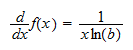(remember that).

## Derivative of an exponential function

Ifis the exponential functionthen its first derivative isIf the exponential functiondoes not have the natural base, but another positive base, that is, ifthen its first derivative is(remember that).

## Derivative of a linear combination of functions

Ifandare two functions andare two constants, then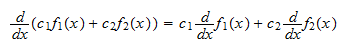In other words, the derivative of a linear combination is equal to the linear combinations of the derivatives. This property is called "linearity of the derivative".

Two special cases of this rule are## Derivative of a product of functions

Ifandare two functions, then the derivative of their product is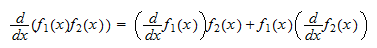## Derivative of a composition of functions (chain rule)

Ifandare two functions, then the derivative of their composition isWhat does this chain rule mean in practice? It means that first you need to compute the derivative of:Then, you substitutewith:Finally, you multiply it by the derivative of:## Derivatives of trigonometric functions

The trigonometric functions have the following derivatives:while the inverse trigonometric functions have the following derivatives: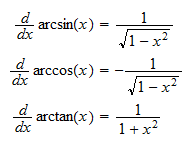## Derivative of an inverse function

Ifis a function with derivativethen its inversehas derivative